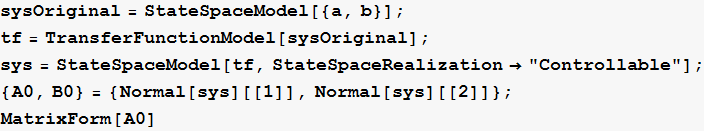Pole assignment state feedback design example using Mathematica

Nasser M. Abbasi
Nov 29, 2014

Detailed step by step showing how to design a gain vector K for single input system in state space such that the system has desired pole locations.

Define the A and B matrices for state space  x’=Ax+Buconvert to controllable form (we call this the target system)Obtain controllability matrix for original systemObtain controllability matrix for target systemObtain T, the transformation matrixVerify Tdefine the gains as unknowns. These are what we will determine using pole assignmentgenerate the closed loop state feedback A matrix using the traget A,B systemFind the CharacteristicPolynomial of the above matrixExtract the coefficients so we can compare them to the design polynomial belowGenerate the design polynomial from the desired pole locationsExtract the coefficients of the design polynomialSolve for the gains by comparing coefficients of design polynomial with the closed loop polynomialConvert the gain vector to original space using the transformation T found aboveverify the original space now has designed eigenvalues with this gain vectorUsing packaged function for design

The above design is implemented in my package now using the function nma`getStateGainVector. Here are examples using itAnother exampleTurn on the flag to see the steps made in the design